## 6.4.2 Cylindrical Algebraic Decomposition

Cylindrical algebraic decomposition is a general method that produces a cylindrical decomposition in the same sense considered in Section 6.3.2 for polygons in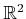and also the decomposition in Section 6.3.4 for the line-segment robot. It is also referred to as Collins decomposition after its original developer [40,232,233]. The decomposition in Figure 6.19 can even be considered as a cylindrical algebraic decomposition for a semi-algebraic set in which every geometric primitive is a linear polynomial. In this section, such a decomposition is generalized to any semi-algebraic set in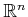.

The idea is to develop a sequence of projections that drops the dimension of the semi-algebraic set by one each time. Initially, the set is defined over, and after one projection, a semi-algebraic set is obtained in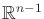. Eventually, the projection reaches, and a univariate polynomial is obtained for which the zeros are at the critical places where cell boundaries need to be formed. A cell decomposition of-cells (intervals) and 0-cells is formed by partitioning. The sequence is then reversed, and decompositions are formed fromup to. Each iteration starts with a cell decomposition in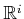and lifts it to obtain a cylinder of cells in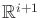. Figure 6.35 shows how the decomposition looks for the gingerbread example; since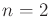, it only involves one projection and one lifting.

Subsections
Steven M LaValle 2012-04-20